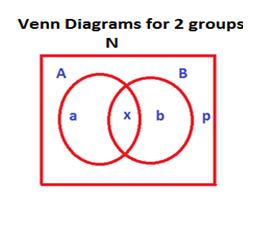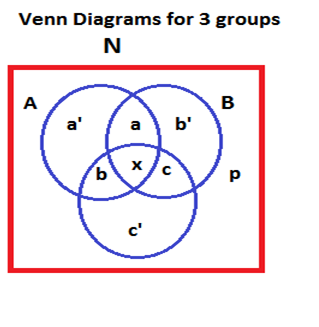## Venn Diagram/Overlapping Sets

Venn diagrams is a convenient way of representing data

We will discuss below representing data using the method of Venn diagrams for 2 groups and 3 groups:

First,From the above figure, consider the following data:

The box denotes a class having N students. The N students are divided as below:

Number of students in one group is A

Number of students in another group is B,

Number of students in both the groups is x,

Number of students who are in neither A nor B is p,

Now, the following formula arise from the above data i.e. using venn diagrams for 2 groups:

Number of students in:

1. At least one group

(i.e., number of students who belong to any one group or either A or B) is:

A + B – x

2. Only one group

(i.e. in only A or in only B) is:

A + B – 2x

3. Total strength of the class:

(including those who belong to any one group and also none of the two groups) is

A + B – x + p, which N is, i.e.,

N = A + B – x + p

Let us solve a question to illustrate the above concept of Venn diagrams used to represent data comprising 2 groups:

Question:

In a class of 100 students, 50 like singing, 35 painting and 25 like neither singing nor painting. How many like both the activities?

Solution:

In the data given, take:

N as 100, A as 50, B as 35 and p as 25.

What needs to be found is x, i.e., those who like both singing and painting.

Apply N = A + B – x + p

100 = 50 + 35 – x +25,

On solving the above equation, p = 10

Therefore, in the class, 10 like either singing or painting,In the above group, the respective terms (letters) denote the following data:

Number of students in:

One group is A,

a 2nd group is B,

a third group is C,

all three groups is x,

A or B but not C is a,

A or C but not A is b,

B or C but not A is c,

a', b’ and c’ denote those who belong to only A, only B and only C but not the other two groups in each respectively.

From the data as constructed in the above Venn diagram, the following formulae arise:

Number of students who belong to

1. At least one group is

A + B + C – (a + b + c +2x)

2. Only two groups but not the third group is

a + b + c

3. The entire class of N students who belong to any one group or none of the three groups is

N = A + B + C – (a + b + c + 2x) +p

(p denotes number of students who do  not belong to any group)

Let us solve a question applying the above formula:

Question:

In a class of 90 students, 50 can speak English; 40 german; and 35 french. 10 students can speak all of the three languages. How many of the students can speak only any of the two languages?

Solution:

Apply the third formula above for 3-group Venn diagrams:

N = A + B + C – (a + b + c + 2x) + p

What is required is a + b + c

90 = 50 + 40 + 35 – ((a + b + c) + 2 * 10)

a + b + c = 15

Therefore, 15 students can speak any of only two languages.